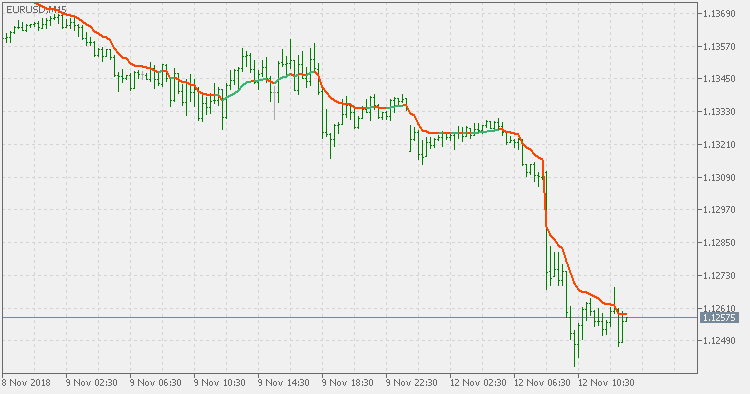Interesting script?
So post a link to it -
let others appraise it

You liked the script? Try it in the MetaTrader 5 terminal# Range weighted EMA - indicator for MetaTrader 5

Views:
1414
Rating:
Published:
2018.11.12 12:35
Updated:
2019.01.29 16:26

Theory:

Range weighted exponential moving average (EMA) is, unlike the "regular" range weighted average (originally published here : Range Weighted Average) calculated in a different way. Even though the basis - the range weighting - is the same, the way how it is calculated is completely different. By definition this type of EMA is calculated as a ratio of EMA of price*weight / EMA of weight. And the results are very different and the two should be considered as completely different types of averages. The higher than EMA to price changes responsiveness when the ranges increase remains in this EMA too and in those cases this EMA is clearly leading the "regular" EMA.

Usage:

You can use it as any other average (including the usage of the color change as signals).Range weighted average MACD

Range weighted average MACDRange weighted average

Range weighted average - average that uuse the current volatility for weighted average calculationRange weighted smoothed EMA

Range weighted smoothed EMARocket RSI

Rocket RSI